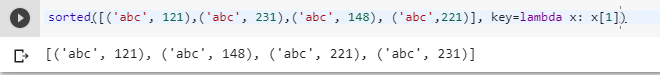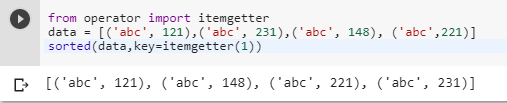# Sort a list of tuples by 2nd item (integer value)

0 votes
1 view
in Python

I have a list of tuples that looks something like this:

[('abc', 121),('abc', 231),('abc', 148), ('abc',221)]

I want to sort this list in ascending order by the integer value inside the tuples. Is it possible?

## 1 Answer

0 votes
by (107k points)

If you want to sort a list of tuples by 2nd item in ascending order you can do by using the sorted function. Below is the code for the same which shows how to do it.

sorted([('abc', 121),('abc', 231),('abc', 148), ('abc',221)], key=lambda x: x)In the above code, the key is a function that identifies how to retrieve the comparable element from your data structure. So you are using the second element of the tuple.

Another good way of doing this by using the itemgetter function.

from operator import itemgetter

data = [('abc', 121),('abc', 231),('abc', 148), ('abc',221)] sorted(data,key=itemgetter(1))0 votes
1 answer
0 votes
1 answer
0 votes
1 answer
0 votes
1 answer
0 votes
1 answer